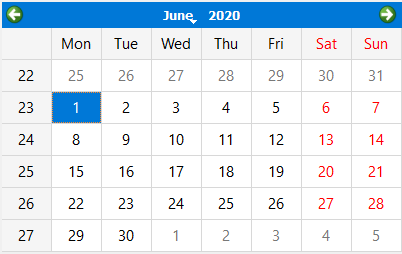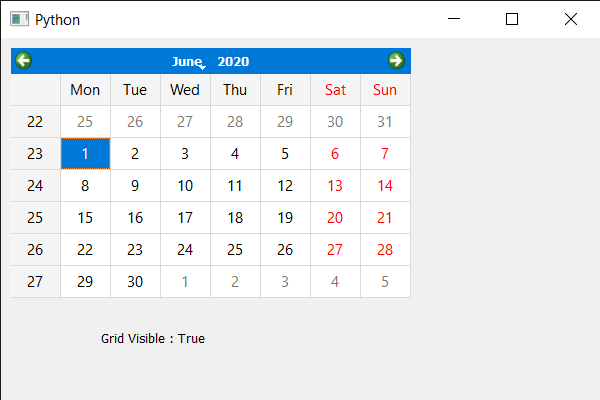# PyQt5 QCalendarWidget – Checking if grid is visible

• Last Updated : 27 Jul, 2021

In this article, we will see how we can check if grid is visible in the QCalendarWidget. Grid is basically the inter connected network of lines that separates the dates with each other. By default, there is no grid to the QCalendarWidget although we can set grid any time with the help of setGridVisible method. Below is how calendar with grid looks like.In order to do this we will use isGridVisible method with the QCalendarWidget object.
Syntax : calendar.isGridVisible()
Argument : It takes no argument
Return : It return bool

Below is the implementation

## Python3

 `# importing libraries``from` `PyQt5.QtWidgets ``import` `*``from` `PyQt5 ``import` `QtCore, QtGui``from` `PyQt5.QtGui ``import` `*``from` `PyQt5.QtCore ``import` `*``import` `sys`  `class` `Window(QMainWindow):` `    ``def` `__init__(``self``):``        ``super``().__init__()` `        ``# setting title``        ``self``.setWindowTitle(``"Python "``)` `        ``# setting geometry``        ``self``.setGeometry(``100``, ``100``, ``600``, ``400``)` `        ``# calling method``        ``self``.UiComponents()` `        ``# showing all the widgets``        ``self``.show()` `    ``# method for components``    ``def` `UiComponents(``self``):` `        ``# creating a QCalendarWidget object``        ``calendar ``=` `QCalendarWidget(``self``)` `        ``# setting geometry to the calendar``        ``calendar.setGeometry(``10``, ``10``, ``400``, ``250``)` `        ``# setting grid``        ``calendar.setGridVisible(``True``)` `        ``# creating label``        ``label ``=` `QLabel(``self``)` `        ``# setting geometry to the label``        ``label.setGeometry(``100``, ``270``, ``250``, ``60``)` `        ``# making label multi line``        ``label.setWordWrap(``True``)` `        ``# checking if the calendar has grid``        ``check ``=` `calendar.isGridVisible()` `        ``# setting text to the label``        ``label.setText(``"Grid Visible : "` `+` `str``(check))`  `# create pyqt5 app``App ``=` `QApplication(sys.argv)` `# create the instance of our Window``window ``=` `Window()` `# start the app``sys.exit(App.``exec``())`

Output:My Personal Notes arrow_drop_up